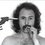# Explain to me how this works

If two point charges are separated by distance then according to Coulomb's law, the attractive force between them is fve = kvexq^2/d^2...

where is Coulomb's constant. But what if the charges were distributed on spheres instead of at points? Two charged conducting spheres with charges and are separated by center-to-center distance

The attractive force between them is ?

the answer to this question in the weekly is plugging in the answers to the question. the attractive force between is equal to the equation, but the answer is wrong and say the attractive for is greater than. so explain to me. how fve = kvexq^2/d^2 =/< fve

this is illogical

if the force was greater than, the distance would be decreasing, right? less than, and they would be increasing in distance because + and - q would want to balance out.

im guessing kve is the matter or space bettween them, medium between them or resistance. which confuses me because it's multiplied.

maybe im just really stupid, but as far as i know how equations work. equal to is equal to and not great than or less than.Note by Bo Treat
3 years, 4 months ago

This discussion board is a place to discuss our Daily Challenges and the math and science related to those challenges. Explanations are more than just a solution — they should explain the steps and thinking strategies that you used to obtain the solution. Comments should further the discussion of math and science.

When posting on Brilliant:

• Use the emojis to react to an explanation, whether you're congratulating a job well done , or just really confused .
• Ask specific questions about the challenge or the steps in somebody's explanation. Well-posed questions can add a lot to the discussion, but posting "I don't understand!" doesn't help anyone.
• Try to contribute something new to the discussion, whether it is an extension, generalization or other idea related to the challenge.

MarkdownAppears as
*italics* or _italics_ italics
**bold** or __bold__ bold
- bulleted- list
• bulleted
• list
1. numbered2. list
1. numbered
2. list
Note: you must add a full line of space before and after lists for them to show up correctly
paragraph 1paragraph 2

paragraph 1

paragraph 2

[example link](https://brilliant.org)example link
> This is a quote
This is a quote
    # I indented these lines
# 4 spaces, and now they show
# up as a code block.

print "hello world"
# I indented these lines
# 4 spaces, and now they show
# up as a code block.

print "hello world"
MathAppears as
Remember to wrap math in $$ ... $$ or $ ... $ to ensure proper formatting.
2 \times 3 $2 \times 3$
2^{34} $2^{34}$
a_{i-1} $a_{i-1}$
\frac{2}{3} $\frac{2}{3}$
\sqrt{2} $\sqrt{2}$
\sum_{i=1}^3 $\sum_{i=1}^3$
\sin \theta $\sin \theta$
\boxed{123} $\boxed{123}$

Sort by:

say the attractive for is greater than. so explain to me. how fve = kvexq^2/d^2 =/< fve

The important point is that the spheres are conducting, so charge can freely move on the sphere. The charge arrange such that the potential is minimized, so the effective distance is less than $d$, say $d_{sphere} < d$, so the force is now $\dfrac{kq^2}{d_{sphere}^2} > \dfrac{kq^2}{d^2}$.

if the force was greater than, the distance would be decreasing, right? less than, and they would be increasing in distance because + and - q would want to balance out.

The question asks for the force at one instant. Sure, the spheres attract each other because they have opposite signs, and will start moving towards each other.

- 3 years, 3 months ago